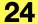## API_​AnalyticalCurveType

Describes the type of the Structural Analytical Model Curve.

```    typedef enum {
API_AnalyticalCurve_LineType,
API_AnalyticalCurve_ArcType
API_AnalyticalCurve_SplineType
API_AnalyticalCurve_EllipseType
} API_AnalyticalCurveType;
```

#### Members

API_AnalyticalCurve_LineTypeThe Structural Analytical Model Curve is a simple line segment.
API_AnalyticalCurve_ArcTypeThe Structural Analytical Model Curve is an arc segment.
API_AnalyticalCurve_SplineTypeThe Structural Analytical Model Curve is a spline multi point segment.
API_AnalyticalCurve_EllipseTypeThe Structural Analytical Model Curve is an ellipse arc segment.

#### Requirements

Version: API 24 or later